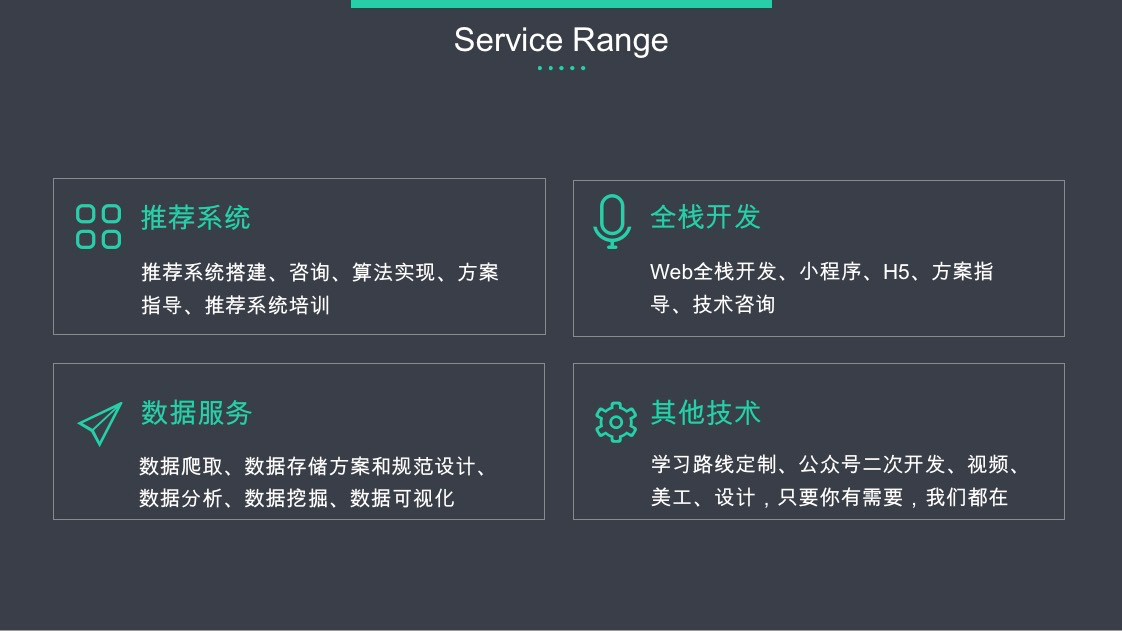# 高数中的导数

1）点导数是因变量在$x_0$ 处的变化率，它反映了因变量随自变量的变化而变化的快慢成都

2）如果函数y = f(x)在开区间 I 内的每点都可导，就称f(x)在开区间 I 内可导

3）对于任一 x 属于 I ，都对应着函数f(x)的一个导数，这个函数叫做原来函数f(x)的导函数

4）导函数在x1 处 为 0，若 x<1 时，f’(x) > 0 ，这 f(x) 递增，若f’(x)<0 ，f(x)递减

5）f’(x0) 表示曲线y=f(x)在点 （x0,f($x_0$)）处的切线斜率

# 偏导数

$z_x(x=1,y=2) = 8$
$z_y(x=1,y=2) = 7$

# 梯度

1）梯度是一个向量
2）梯度的方向是最大方向导数的方向
3）梯度的值是最大方向导数的值

# 梯度下降与梯度上升

## 梯度下降

### 关于梯度下降的几个概念

1）步长（learning rate）：步长决定了在梯度下降迭代过程中，每一步沿梯度负方向前进的长度
2）特征（feature）：指的是样本中输入部门，比如样本（x0，y0），（x1，y1），则样本特征为x，样本输出为y
3）假设函数（hypothesis function）：在监督学习中，为了拟合输入样本，而使用的假设函数，记为$h_θ(x)$。比如对于样本$（x_i,y_i）(i=1,2,…n)$,可以采用拟合函数如下： $h_θ(x) = θ0+θ1_x$。
4）损失函数（loss function）：为了评估模型拟合的好坏，通常用损失函数来度量拟合的程度。损失函数极小化，意味着拟合程度最好，对应的模型参数即为最优参数。在线性回归中，损失函数通常为样本输出和假设函数的差取平方。比如对于样本（xi,yi）(i=1,2,…n),采用线性回归，损失函数为：

### 梯度下降的代数方法描述

1. 先决条件：确定优化模型的假设函数和损失函数
这里假定线性回归的假设函数为$h_\theta(x_1,x_2,…x_n)=\theta_0+\theta_1x_1+…+\theta_nx_n$，其中 $\theta _i(i=0,1,2…n)$ 为模型参数(公式中用$\theta$代替)，$x_i(i=0,1,2…n)$为每个样本的n个特征值。

则对应选定得损失函数为：

2. 算法相关参数的初始化
主要是初始化 $\theta _0,\theta _1…,\theta _n$，算法终止距离 $\varepsilon$ 以及步长 $\alpha$。在没有任何先验知识的时候，我喜欢将所有的 $\theta$ 初始化为0， 将步长初始化为1。在调优的时候再优化。

3. 算法过程

• 1)：确定当前损失函数的梯度，对于$\theta _i$，其梯度表达式为：

• 2)：用步长乘以损失函数的梯度，得到当前位置的下降距离，即

• 3)：确定是否所有的$\theta _i$ ，梯度下降的距离都小于 $\varepsilon$，如果小于$\varepsilon$，则算法停止，当前所有的 $\theta _i(i=1,2,3,…,n)$ 即为最终结果。否则执行下一步。

• 4)：更新所有的 $\theta$，对于$\theta _i$，其更新表达式如下。更新完毕后继续转入步骤1)。

### 梯度下降的矩阵方式描述

1. 先决条件：确定优化模型的假设函数和损失函数
这里假定线性回归的假设函数为$h_\theta(x_1,x_2,…x_n)=\theta_0+\theta_1x_1+…+\theta_nx_n$，其中 $\theta _i(i=0,1,2…n)$ 为模型参数，$x_i(i=0,1,2…n)$为每个样本的n个特征值。
假设函数对应的矩阵表示为：$h_\theta (x) = X \theta$，假设函数 $h_\theta(x)$ 为mx1的向量，$\theta$ 为nx1的向量，里面有n个代数法的模型参数。X为mxn维的矩阵。m代表样本的个数，n代表样本的特征数。
则对应选定得损失函数为：其中YY是样本的输出向量，维度为m*1

2.算法相关参数初始化:
$\theta$ 向量可以初始化为默认值，或者调优后的值。算法终止距离 $\varepsilon$ ，步长 $\alpha$ 和 “梯度下降的代数方法”描述中一致。

3.算法过程
• 1)：确定当前位置的损失函数的梯度，对于 $\theta$ 向量,其梯度表达式如下：

• 2)：用步长乘以损失函数的梯度，得到当前位置下降的距离，即 $\alpha \frac{ \partial }{\partial \theta } \jmath (\theta)$
• 3)：确定 $\theta$ 向量里面的每个值,梯度下降的距离都小于 $\varepsilon$，如果小于 $\varepsilon$ 则算法终止，当前 $\theta$ 向量即为最终结果。否则进入步骤4)
• 4)：更新 $\theta$ 向量，其更新表达式如下。更新完毕后继续转入步骤1)

## 梯度下降的算法优化

1. 算法的步长选择。在前面的算法描述中，我提到取步长为1，但是实际上取值取决于数据样本，可以多取一些值，从大到小，分别运行算法，看看迭代效果，如果损失函数在变小，说明取值有效，否则要增大步长。前面说了。步长太大，会导致迭代过快，甚至有可能错过最优解。步长太小，迭代速度太慢，很长时间算法都不能结束。所以算法的步长需要多次运行后才能得到一个较为优的值。

2. 算法参数的初始值选择。 初始值不同，获得的最小值也有可能不同，因此梯度下降求得的只是局部最小值；当然如果损失函数是凸函数则一定是最优解。由于有局部最优解的风险，需要多次用不同初始值运行算法，关键损失函数的最小值，选择损失函数最小化的初值。

3.归一化。由于样本不同特征的取值范围不一样，可能导致迭代很慢，为了减少特征取值的影响，可以对特征数据归一化，也就是对于每个特征x，求出它的均值 $\bar{x}$ 和标准差std(x)，然后转化为：

http://blog.csdn.net/walilk/article/details/50978864

https://www.zhihu.com/question/24658302

https://www.cnblogs.com/pinard/p/5970503.html

http://www.doc88.com/p-7844239247737.html

【技术服务】，详情点击查看： https://mp.weixin.qq.com/s/PtX9ukKRBmazAWARprGIAg